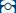+49 9135 73666 65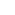0# What are the most important quality characteristics for switching power supplies?

selection criterion Properties
Low cost Low initial cost, Low operating cost due to high efficiency and long service life
High efficiency Efficiency >90%: Reduces operating costs in the long term
High lifetime, reliability High MTBF >800,000 hrs.
Conformal coating is also applied on the PCBAs to provide protection against dust and chemical pollutants
Good EMC Radiation characteristics according to EN55022 and immunity to radiation and interference according to EN61000, PFC
Low power dissipation (energy cost + heat dissipation) + EMC Active PFC - power factor correction: reduces harmonic content and energy losses
Quality of voltage and current (e.g. voltage accuracy, step response, harmonics, control dynamics, etc.) Power Boost - power reserve
Auto Recovery function
Safety Protection against electric shock, OVP, OTP, short circuit proof
Good temperature performance Applicability at bes. high or low temp. e.g. -20°C to +80°C
Low size - volume High power density
Low weight (esp. for mobile applications)

## 1. cost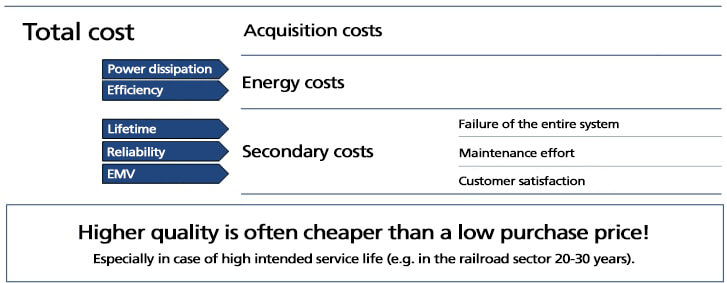## 2. efficiency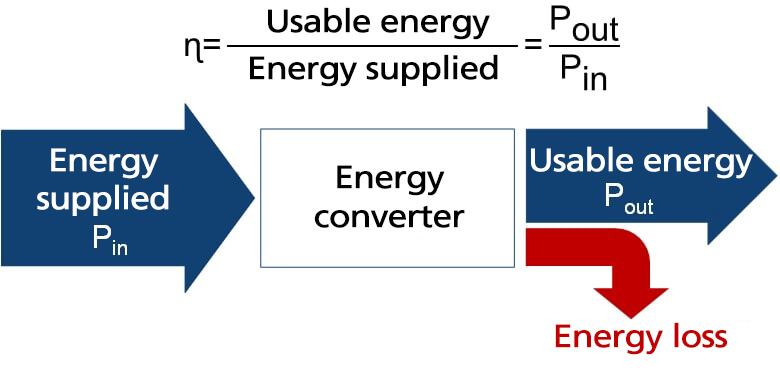High efficiency is achieved by:

• Sophisticated circuit technology
• High-quality components - e.g. switching transistors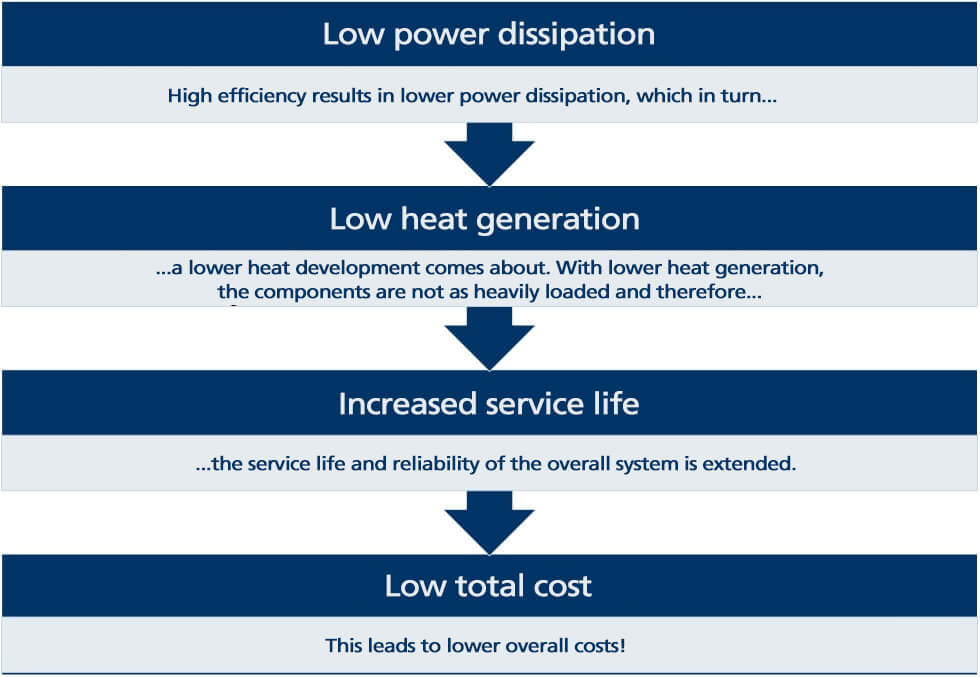## 3. Power factor - PFC

### What is "power factor"?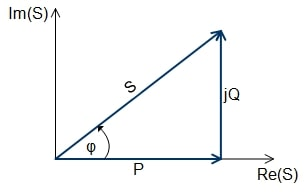The power factor is the ratio between active (P) and apparent (S) power.
 Power factor = λ = |P| S
• Active power P: power transmitted to the output
• Apparent power S: power drawn from the grid
A high power factor represents an effective use of electrical energy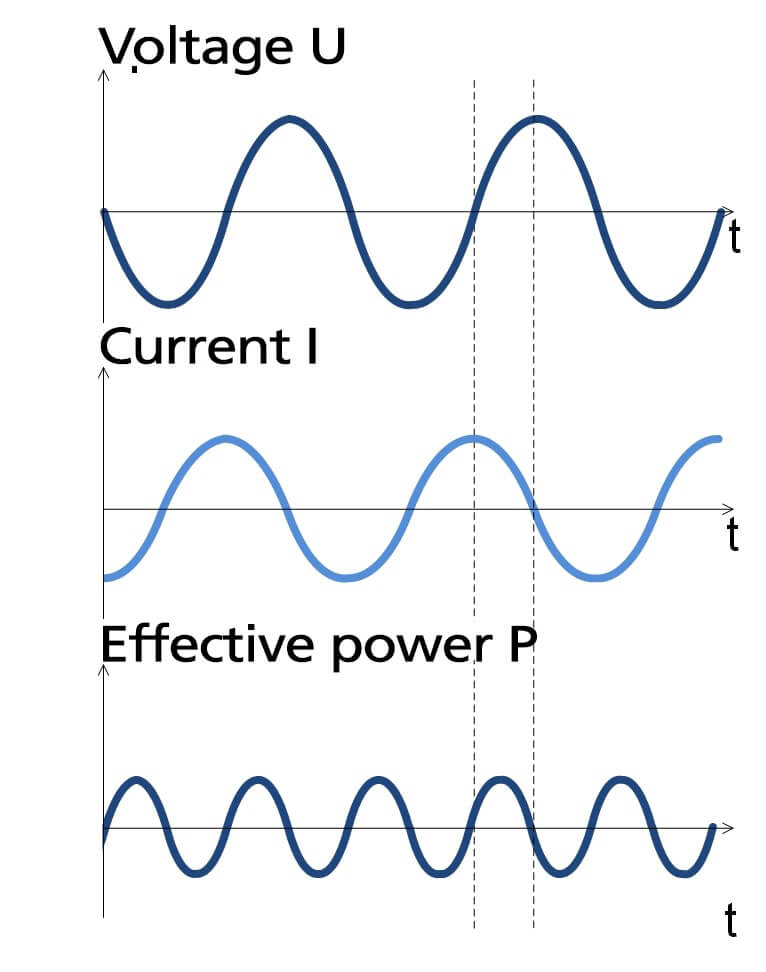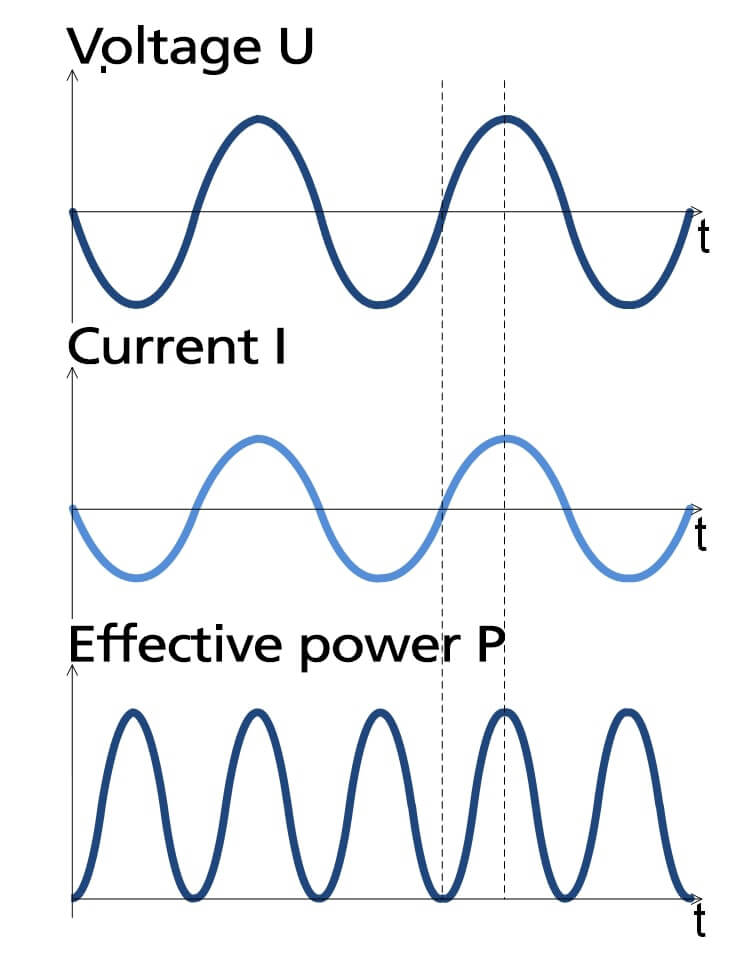If there is a phase shift of voltage and current due to impedances (Fig. 2), the energy continuously oscillates between the generator and the electrical load, putting a load on the power system and the generating equipment.

As shown in Figure 3, the active power is maximum when the current and voltage are in phase. Then the active power is equal to the apparent power and the power factor is equal to one.

### Why do I need a PFC in the switching power supply?

PFC stands for Power Factor Correction.

As previously described, a high power factor represents an effective use of electrical energy. Therefore a phase equality between current and voltage, as well as as ideal as possible sinusoidal curves is to be striven for in principle.

A simple phase shift between current and voltage due to linear impedances are rather rarely the case. In the case of a linear inductive load, for example, a simple capacitor can be connected in parallel to effect power factor correction.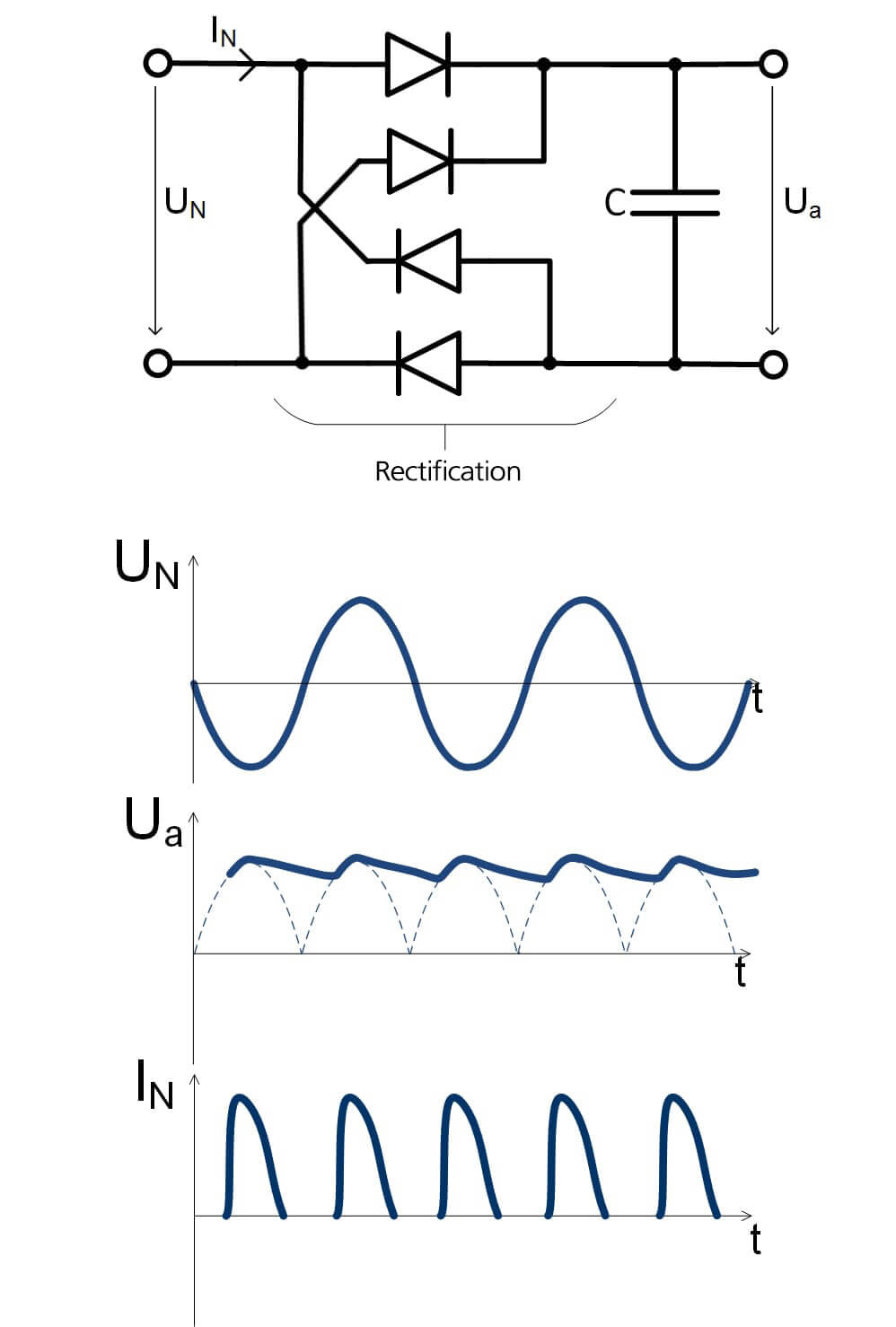Significantly more often, however, consumers are non-linear and no longer draw their current from the public power supply network in a sinusoidal manner as in the past, but in a pulse-shaped manner. Cause for this is the ever further spread of devices, which represent electronically regulated loads.

A switching power supply is such a typical nonlinear load. In its simplest form, the power converter consists of an input circuit with a half-wave or full-wave rectifier, followed by a storage capacitor to provide an unregulated DC voltage (intermediate circuit voltage). This topology draws current only when the line voltage is greater than the DC link voltage, i.e., only at the peaks of the line voltage, so that the filter capacitor is recharged in pulses via the rectifier diodes. Each pulse contains enough energy to supply the load until the next voltage peak (Fig. 4).

The resulting output current is highly distorted compared to the sinusoidal input and contains a number of harmonics.

The phase shift between current and voltage creates reactive power that utilities can only handle by oversizing all components in the utility grid. To prevent this, the EU first initiated the EN60555-2 standard ("Reactions in power supply systems caused by household appliances and by similar electrical equipment. Part 2: Harmonics") and, from 2001, the European standard EN61000-3-2 ("Electromagnetic compatibility (EMC); Part 3: Limits; Section 2: Limits for harmonic currents (equipment input current < 16A per conductor)"). This prescribes a limit on the level of harmonic currents from the mains for all equipment with a power consumption of more than 75W.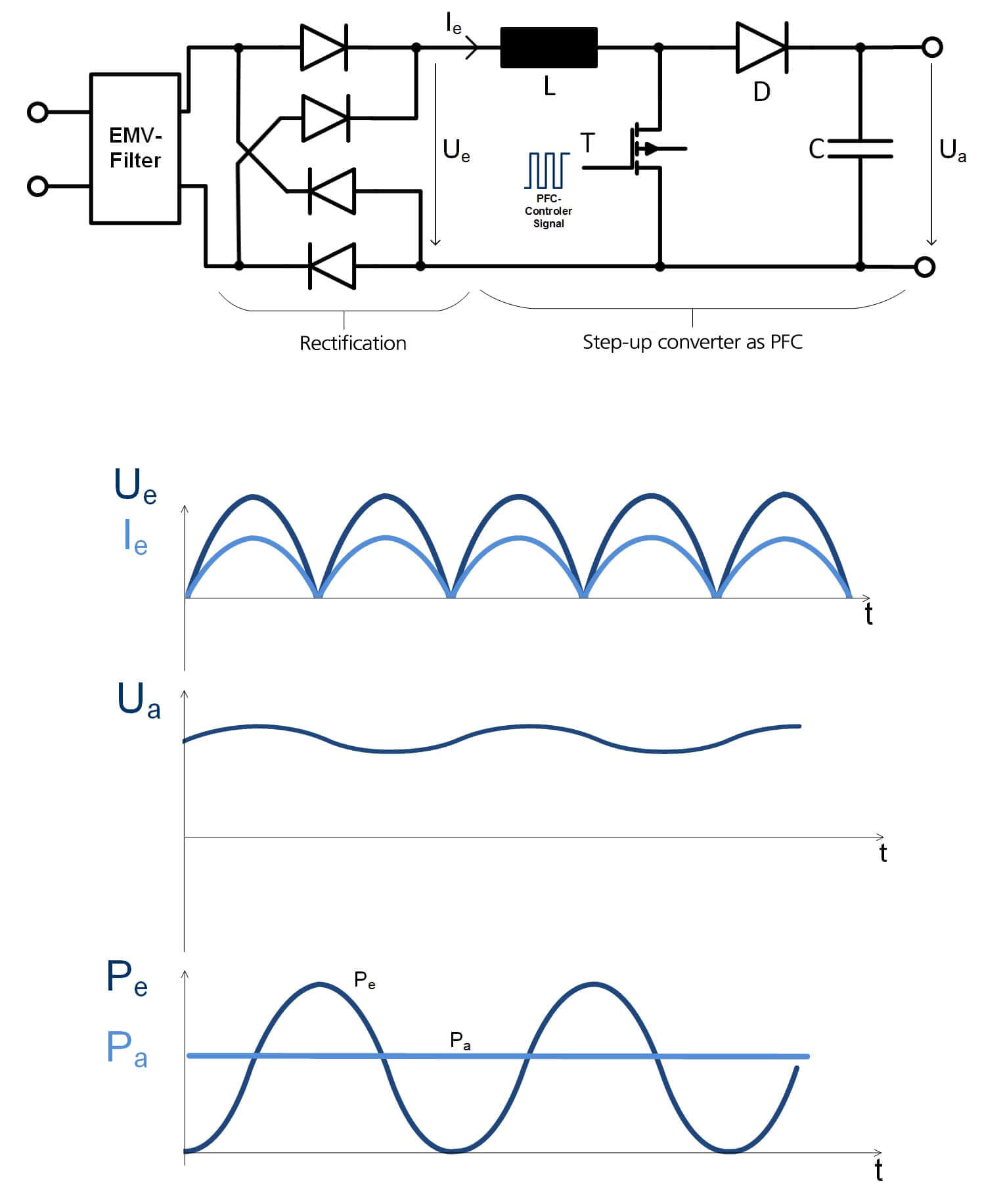These specifications can only be met by correcting the power factor with a PFC.

The PFC extends the current draw from the grid, reducing the magnitude and speed of the rise of the current through the rectifier diodes, which reduces the number and magnitude of the harmonics.

To achieve this, one uses a boost converter (see Fig. 5).

However, the PFC differs from the boost converter in the control.
While the output voltage is converted as usual to a higher than the highest possible input voltage (this is 360V in the European network),
however, the transistor is clocked so that the mains current is almost sinusoidal.
The current in the inductor is managed so that it is proportional to the input voltage Ue.

### The regulation of PFC:

The regulation and control of the switching transistor is performed by various integrated circuits, PFC controllers.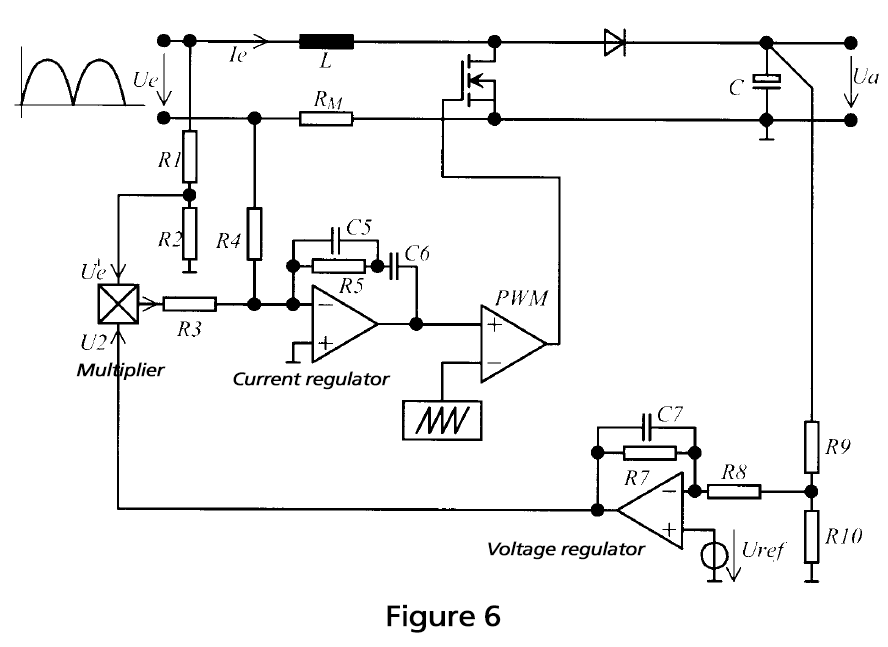Basically, two control loops are needed:

A control loop that adjusts the input current of the PFC Ie proportional to the instantaneous value of the input voltage Ue. This is because only when this current follows the sinusoidal input voltage is the line current also sinusoidal and in phase with the line voltage. This control loop is called a current control loop.

A second control loop adjusts the rms value of the choke current so that the average output voltage Ua of the PFC remains constant despite varying output power. This control loop is called the voltage control loop.

So, in summary:

The rms value of the input current is controlled by the voltage control loop, whereas the current control loop ensures that the input current is sinusoidal.

The active PFC generates additional high-frequency interference, which must be suppressed with an upstream passive line filter.